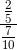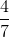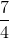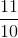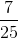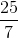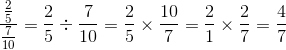# ISEE Middle Level Math : How to divide fractions

## Example Questions

← Previous 1 3 4 5

### Example Question #1 : How To Divide Fractions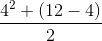Explanation:

In order to solve:

First, solve the exponent equation within the numerator portion of the fraction: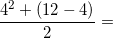Then, solve the equation within the parentheses: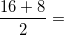Then, solve the entire equation in the numerator portion and divide the numerator by the denominator.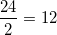### Example Question #2 : How To Divide Fractions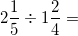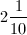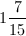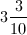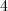Explanation:

To solve:

First, turn the mixed fractions into improper fractions.Then, change the operation to multiplication and flip the second fraction. Solve accordingly and simplify the answer if possible.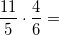The answer is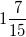.

### Example Question #3 : How To Divide Fractions

What isdivided by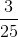?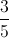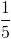Explanation: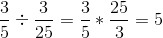### Example Question #4 : How To Divide Fractions

Evaluate: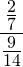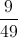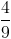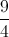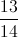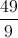Explanation:

Rewrite this horizontally as a division expression, rewrite as a product, cross-cancel, and multply across: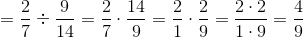### Example Question #1 : How To Divide Fractions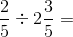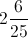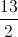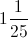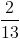Explanation:

First, convert each fraction into an improper fraction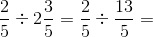Then, change the operation to mulitplication and flip the second fraction: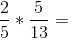Reduce and solve accordingly:

Answer: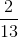### Example Question #6 : How To Divide Fractions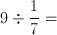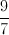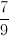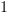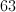Explanation:

We never actually divide fractions.  When given a division problem, you multiply by the reciprocal of the 2nd fraction.

So,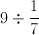becomes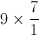.

This is the same as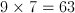### Example Question #7 : How To Divide Fractions

Divide: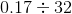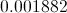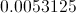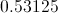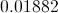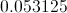Explanation:

Simply use the long division process. The quotient will be: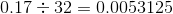### Example Question #8 : How To Divide Fractions

Solve: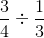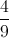Explanation: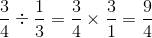### Example Question #9 : How To Divide Fractions

Simplify: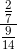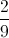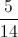Explanation:

Rewrite this as a division, dividing the numerator by the denominator: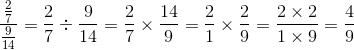### Example Question #10 : How To Divide Fractions

Simplify: